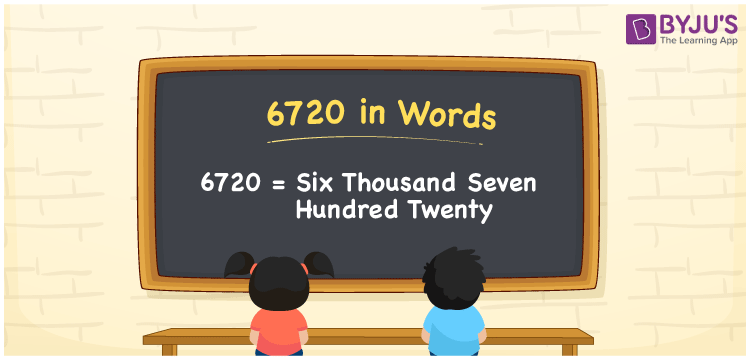# 6720 in Words

We can express 6720 in words as Six Thousand Seven Hundred Twenty. For example, Kavita saved Rs. 6720 in 3 months, then you can express the same sentence in words as “Kavita saved Rupees Six Thousand Seven Hundred Twenty in three months”. 6720 is a cardinal number as it denotes a specific quantity. Let us learn some interesting facts on the number 6720 along with conversion into words in this article.

 6720 in Words Six Thousand Seven Hundred Twenty Six Thousand Seven Hundred Twenty in numerical form 6720

## 6720 in English Words

Generally, we express the numbers in words using the English alphabet. Thus, we can read the number 6720 in English as Six Thousand Seven Hundred Twenty.## How to Write 6720 in Words?

6720 is a four-digit number. So, let us create a chart of 4 columns to represent the place value chart for the number 6720. This can be done as shown below.

 Thousands Hundreds Tens Ones 6 7 2 0

Hence, we can write the expanded form as:

6 x Thousand + 7 x Hundred + 2 x Ten + 0 x One

= 6 x 1000 + 7 x 100 + 2 x 10 + 0 x 1

= 6000 + 700 + 20 + 0

= 6000 + 700 + 20

= 6720

= Six Thousand Seven Hundred Seventy

Therefore, 6720 in words is written as Six Thousand Seven Hundred Twenty

Interesting way of writing 6720 in words

6 = Six

67 = Sixty-Seven

672 = Six Hundred and Seventy-Two

6720 = Six Thousand Seven Hundred Twenty

Thus, the word form of the number 6720 is Six Thousand Seven Hundred Twenty

6720 is a natural number that precedes 6721 and succeeds 6719

• 6720 in words – Six Thousand Seven Hundred Twenty
• Is 6720 an odd number? – No
• Is 6720 an even number? – Yes
• Is 6720 a perfect square number? – No
• Is 6720 a perfect cube number? – No
• Is 6720 a prime number? – No
• Is 6720 a composite number? – Yes

## Frequently Asked Questions on 6720 in Words

### Write 6720 in words.

6720 in words is written as Six Thousand Seven Hundred Twenty.

### What is the value of 7000 – 280?

7000 – 280 = 6720 Therefore, the value of 7000 – 280 is 6720.

### Write Six Thousand Seven Hundred Twenty in numbers.

Six Thousand Seven Hundred Twenty in numbers is 6720.